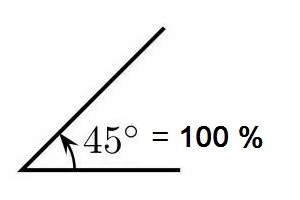Convert the degrees of an angle to percentage of slope with this online calculator.

### Degrees to PercentDegrees:
Input the degrees of an angle to convert to percentage of slope.
Keep in mind that an error will occur for any slope that is 90 degrees
or greater. The percentage approaches infinity as you near 90 degrees.

### How do you convert degrees to percent?

To find the surface area of a cube, use the equation:
Cube

To convert, use this angle degrees to percent of slope formula:
Percentage = [ Tan ( Degrees ) ] x 100

Example: An angle of 48 degrees would be:

Percentage of Slope = [ Tan ( 48 ) ] x 100

Calculated out this gives a slope percentage 111.06 percent because
the rise is greater than the amount of run by 11.06 percent.